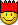# "geometry" autoit help?

## Recommended Posts

So basically this is it, im trying to program a simple game.

There is a graph with a circle located on it. Its center is at the origin, and its radius is any number you want. lets call it x.

There is a radius drawn from the center to any random point on the circle. for this example, lets say the radius is 8, and the intersection is at

(6, 2*sqrt7)

The second intersection is at (0,8)

How would one find out the degrees (or distance) along the circle from the first point, to the second?

##### Share on other sites

You can use trigonemetric ratios to find the degrees. Cos(X) = 6/8. X is your degrees.

Edited by dantay9
##### Share on other sites

Well. If you want a more long winded method than dantays, try this out.

We have a triangle where

Point a = 0,0

Point b = 6, sqrt (11)

Point c = 0, 8

from there we know the sides

c = sqrt (6^2 + sqrt (11) ^ 2) = sqrt (36 + 11) = sqrt (47)

b = 8

a = sqrt (6^2 + (8 - sqrt (11)) ^ 2) = sqrt (36 + (8 - sqrt (11)) ^ 2)

We know know all the sides of a triangle. Use my very nice triangle calculator (or the cosine rule). you should get

A = 61.07°

B = 66.91°

C = 52.02°

If you want the maths...

Cosine rule:

cos A = (b^2 + c^2 - a^2) / 2bc

A = acos( (( 8 ^ 2) ^ 2 + 47 - (36 + (8 - sqrt (11) ^ 2))) / 2 * 8 * sqrt (47))

which gives an errorI give up. but you get the idea! when I am in the mood I'll try to find out where I went wrong.

MDiesel

##### Share on other sites

Well. If you want a more long winded method than dantays, try this out.

We have a triangle where

Point a = 0,0

Point b = 6, sqrt (11)

Point c = 0, 8

from there we know the sides

c = sqrt (6^2 + sqrt (11) ^ 2) = sqrt (36 + 11) = sqrt (47)

b = 8

a = sqrt (6^2 + (8 - sqrt (11)) ^ 2) = sqrt (36 + (8 - sqrt (11)) ^ 2)

We know know all the sides of a triangle. Use my very nice triangle calculator (or the cosine rule). you should get

A = 61.07°

B = 66.91°

C = 52.02°

If you want the maths...

Cosine rule:

cos A = (b^2 + c^2 - a^2) / 2bc

A = acos( (( 8 ^ 2) ^ 2 + 47 - (36 + (8 - sqrt (11) ^ 2))) / 2 * 8 * sqrt (47))

which gives an errorI give up. but you get the idea! when I am in the mood I'll try to find out where I went wrong.

MDiesel

thank you for this, however, i think you guys misunderstood my question. i mean the distance along the circle from point to point.

but its all good, i figured it out, going to use atan for it##### Share on other sites

So basically this is it, im trying to program a simple game.

There is a graph with a circle located on it. Its center is at the origin, and its radius is any number you want. lets call it x.

There is a radius drawn from the center to any random point on the circle. for this example, lets say the radius is 8, and the intersection is at

(6, 2*sqrt7)

The second intersection is at (0,8)

How would one find out the degrees (or distance) along the circle from the first point, to the second?

This appears to work.

```;
\$x1 = 0; First x position
\$x2 = 6; Second x position

#cs
ConsoleWrite(\$ang1*180/(4 * ATan(1)) & @CRLF)
ConsoleWrite(\$ang2*180/(4 * ATan(1)) & @CRLF)

\$deg = abs(\$ang2 - \$ang1)*180/(4 * ATan(1))

; eg. in degrees if angle difference was 90deg, \$ratio would equal 90/360 i.e. 1/4 of the perimeter of the circle
\$ratio = abs(\$ang2 - \$ang1)/(8 * ATan(1)) ; Note (4 * ATan(1)) = Pi

#ce
\$deg = Abs(ACos(\$x2 / \$Radius) - ACos(\$x1 / \$Radius)) * 180 / (4 * ATan(1))
\$ArcLength = 8 * ATan(1) * \$Radius * Abs(ACos(\$x2 / \$Radius) - ACos(\$x1 / \$Radius)) / (8 * ATan(1))
MsgBox(0, "", "Degree difference = " & Round(\$deg, 4) & @CRLF & "Arc length = " & Round(\$ArcLength, 4))
;```

## Create an account

Register a new account

• ### Recently Browsing   0 members

×

• Wiki

• Back

• #### Beta

• Git
• FAQ
×
• Create New...